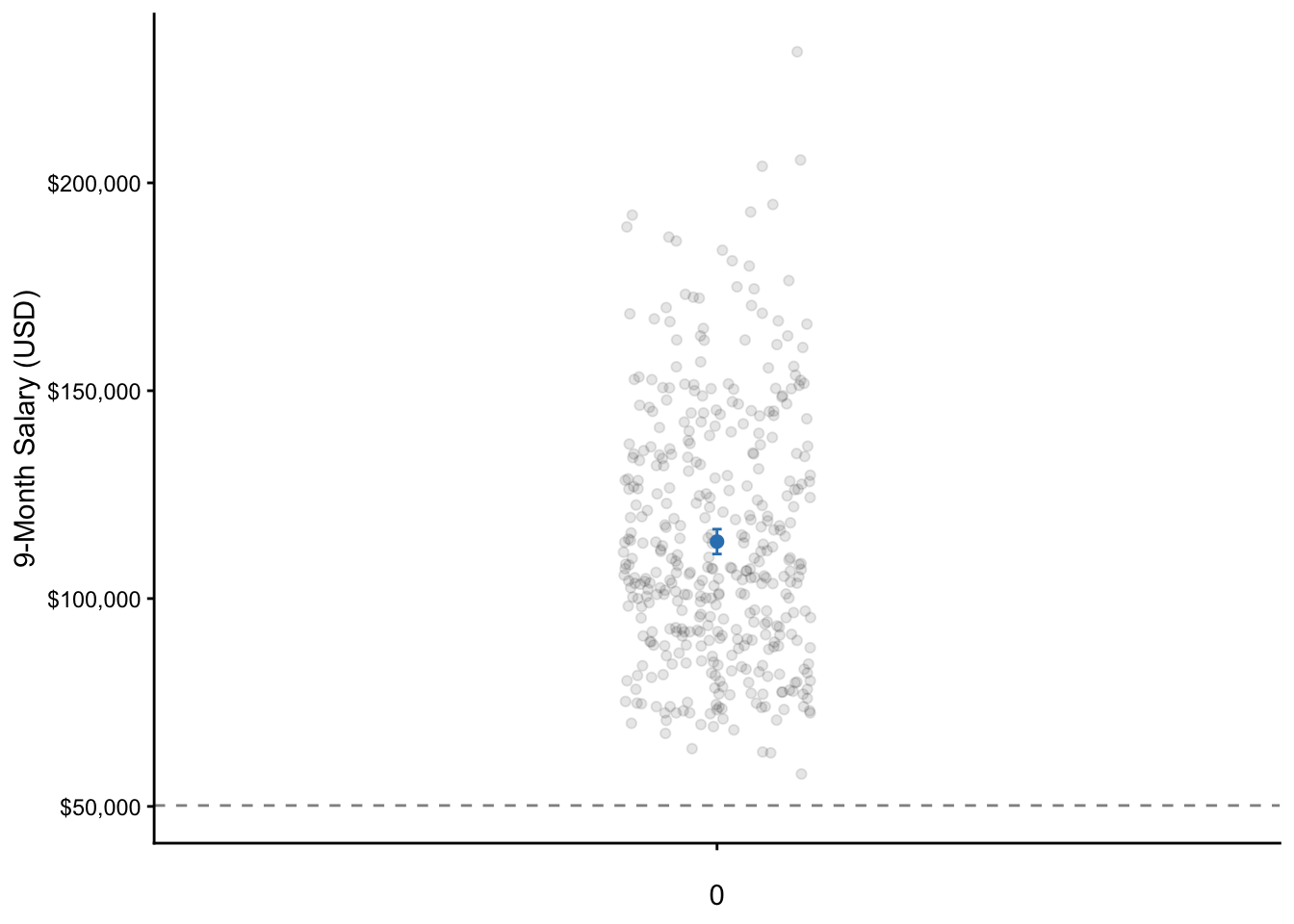# Chapter 7 One Sample t-Test

Now that we have gone over the simple linear regression and how the simple linear regression is written and interpreted identically to the GLM (in addition to its standardized counterpart, the correlation), we can begin covering the other statistical analyses in the order they are typically taught.

Let’s now go over the one-sample t-test, which compares a sample mean to a population mean (or an a priori value).

For example, let’s say we were interested in determining if the salary of professors was significantly different than the national U.S. median income of $50,221 in 2009. For this example, we will again be using the datasetSalaries dataset. ## 7.1 Null and research hypotheses ### 7.1.1 Traditional Approach $H_0: \mu = \50,221$ $H_1: \mu \ne \50,221$ where $$\mu$$ represents the population mean The null hypothesis states that there is no difference between the sample and population mean, or equivalently the sample and population mean are equal. The research hypothesis states that there is a difference between the sample and population mean, or equivalently the sample and population mean are not equal. ### 7.1.2 GLM Approach $Model: Salary = \beta_0 + \varepsilon$ $H_0: \beta_0 = \50,221$ $H_1: \beta_0 \ne \50,221$ where $$\beta_{0}$$ represents the intercept, $$\varepsilon$$ represents the population error, $$H_0$$ represents the null hypothesis, and $$H_1$$ represents the research hypothesis. In this particular case, we will not be using the nil hypothesis as we have an a priori comparison of$50,221.

In the model, when there is no other predictor, the intercept will be the mean. This is because without any other information, the single best number to describe the data is the mean. Thus, the null hypothesis states the intercept (or the mean of 9-month academic salary of professors) is equal to $50,221. The research hypothesis states that the intercept (or the mean 9-month academic salary of professors) is not equal to$50,221.

## 7.2 Statistical analysis

To perform the traditional one-sample t-test, we can use the t.test() function. The first input is the DV of salary, which is again prefixed by the name of the dataset and the dollar sign. The second input is the a priori value (or population value, $$\mu$$) that we are interested in testing (i.e., $50,221). t.test(x = datasetSalaries$salary, mu = 50221)
##
##  One Sample t-test
##

## 7.5 Visualization

# calculate descriptive statistics along with the 95% CI
dataset_summary <- datasetSalaries %>%
dplyr::summarize(
mean = mean(salary),
sd = sd(salary),
n = n(),
sem = sd / sqrt(n),
tcrit = abs(qt(0.05 / 2, df = n - 1)),
ME = tcrit * sem,
LL95CI = mean - ME,
UL95CI = mean + ME
)

# plot
ggplot(datasetSalaries, mapping = aes("", salary)) +
geom_jitter(alpha = 0.1, width = 0.1) +
geom_hline(
yintercept = 50221,
alpha = .5,
linetype = "dashed"
) +
geom_errorbar(
data = dataset_summary,
aes(
y = mean,
ymin = LL95CI,
ymax = UL95CI
),
width = 0.01,
color = "#3182bd"
) +
geom_point(
data = dataset_summary, aes("", mean),
size = 2,
color = "#3182bd"
) +
labs(
x = "0",
y = "9-Month Salary (USD)"
) +
theme_classic() +
scale_y_continuous(labels = scales::dollar)Figure 7.1: A dot plot of the salary of professors where the dot is the mean salary of professors and the whiskers are the 95% CI. Note: The data points are actually only on a single line on the x-axis. They are only jittered (dispersed) for easier visualization of all data points.

1. $$M = \63,486+\50,221=\113,707$$↩︎